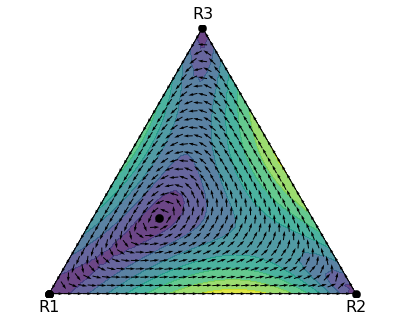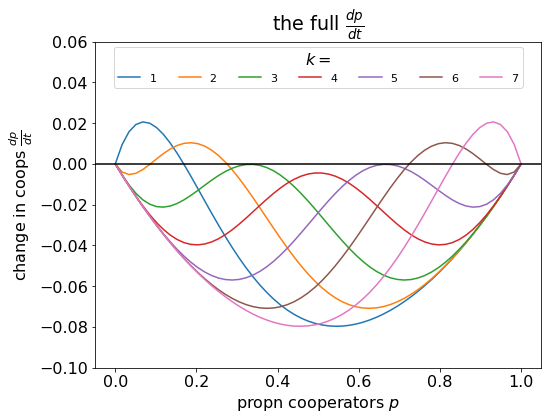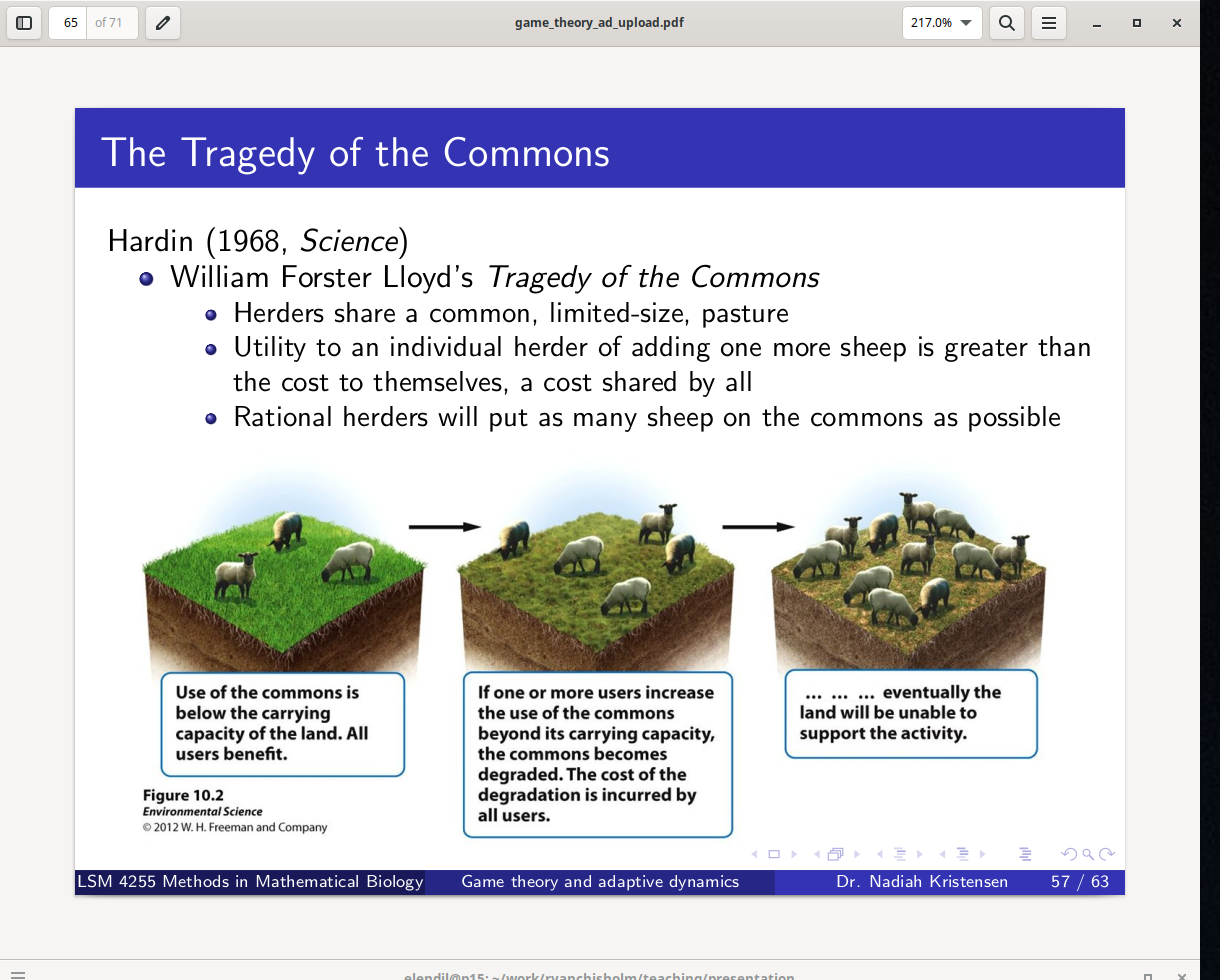# Filed Under #teaching

### Example numerical analysis of replicator dynamics with more than 2 strategiesRecently, I set a task for a student to use Python to analyse the replicator dynamics of a game with three strategies, including using the Jacobian to determine the stability of the steady state. The purpose of this blog post is to share the solution in case that’s useful to someone. The game For this task, we will consider the replicator dynamics of a 2-player game with 3 strategies. Recall the general equation for the replicator dynamics is $\begin{equation} \dot{p}_i = p_i (f_i - \bar{f}) \end{equation}$ where $$p_i$$ is proportion of $$i$$-strategists in the population, $$f_i$$ is fitness effect of...

### Lab meeting about threshold gamesLast month, I chose a paper by Kris De Jaegher in Scientific Reports for our weekly lab-meeting discussion. We used the paper to teach ourselves about threshold games, and the purpose of this blog post is to summarise the things we learnt. Replicator dynamics revision De Jaegher used the replicator dynamics approach. Replicator dynamics assumes a well-mixed, infinitely large population of players that reproduces asexually, where the number of offspring they produce depends upon the payoff they receive from a game-theoretic ‘game’, as illustrated below:– Replicator dynamics animation, created by HowieKor (Creative Commons) We start with a population of $$n$$...

### Lecture notes for LSM4255 Game Theory and Adaptive DynamicsThese are the lecture slides for LSM4255 Game Theory and Adaptive Dynamics. This is the short version with the point-by-point transitions removed. Download: game_theory_ad_upload.pdf Preview of lecture notes.